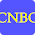# CBSE UGC NET Computer Science Paper II August 2016 (Re-test) - Part 1

1.       The Boolean function [~ (~p˄q)˄~(~p˄~q)]˅(p˄r) is equal to the Boolean function:
(A) q                   (B) p˄r
(C) p˅q              (D) p
2.       Let us assume that you construct ordered tree to represent the compound proposition (~(p˄q))↔(~p˅~q).
Then, the prefix expression and post-fix expression determined using this ordered tree are given as ........... and ............. respectively.
(A) ↔~˄pq˅ ~~pq, pq˄~p~q~˅↔
(B) ↔~˄pq˅ ~p~q, pq˄~p~q~˅↔
(C) ↔~˄pq˅ ~~pq, pq˄~p~ ~q˅↔
(D) ↔~˄pq˅ ~p~q, pq˄~p~~q˅↔
3.       Let A and B be sets in a finite universal set U. Given the following:
|A – B|, |AÅB|, |A|+|B| and |AÈB|
Which of the following is in order of increasing size ?
(A) |A – B| ≤ |AÅB| ≤ |A| + |B| ≤ |AÈB|
(B) |AÅB| ≤ |A – B| ≤ |AÈB| ≤ |A| + |B|
(C) |AÅB| ≤ |A| + |B| ≤ |A – B| ≤ |AÈB|
(D) |A – B| ≤ |AÅB| ≤ |AÈB| ≤ |A| + |B|
4.       What is the probability that a randomly selected bit string of length 10 is a palindrome?
(A) 1/64              (B) 1/32
(C) 1/8                (D) ¼
5.       Given the following graphs :
Which of the following is correct?
(A) G1 contains Euler circuit and G2 does not contain Euler circuit.
(B) G1 does not contain Euler circuit and G2 contains Euler circuit.
(C) Both G1 and G2 do not contain Euler circuit.
(D) Both G1 and G2 contain Euler circuit.

6.       The octal number 326.4 is equivalent to
(A) (214.2)10 and (D6.8)16       (B) (212.5)10 and (D6.8)16
(C) (214.5)10 and (D6.8)16       (D) (214.5)10 and (D6.4)16
7.       Which of the following is the most efficient to perform arithmetic operations on the numbers?
(A) Sign-magnitude    (B) 1’s complement
(C) 2’s complement     (D) 9’s complement
8.       The Karnaugh map for a Boolean function is given as
The simplified Boolean equation for the above Karnaugh Map is
(A) AB + CD + AB’ + AD         (B) AB + AC + AD + BCD
(C) AB + AD + BC + ACD       (D) AB + AC + BC + BCD
9.       Which of the following logic operations is performed by the following given combinational circuit ?
(A) EXCLUSIVE-OR   (B) EXCLUSIVE-NOR
(C) NAND                     (D) NOR
10.    Match the following:
List – I                                    List – II
a. Controlled Inverter              i. a circuit that can add 3 bits
b. Full adder                             ii. a circuit that can add two binary numbers
c. Half adder                             iii. a circuit that transmits a binary word or its
1’s complement
Codes :
a    b    c   d
(A) iii    ii    iv   i
(B) ii     iv   i    iii
(C) iii    iv   i    ii
(D) iii    i    iv   ii
1.